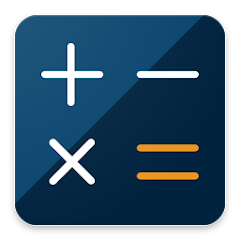1K+Everyone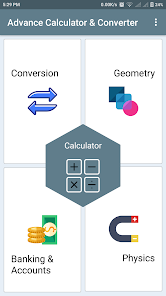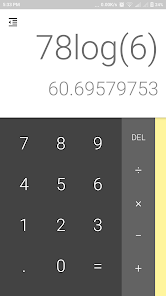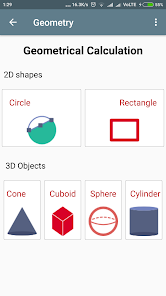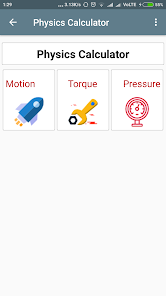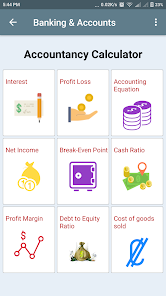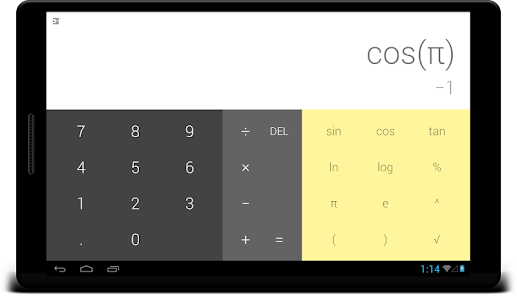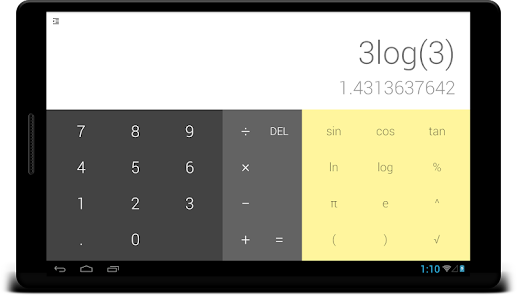The Advance Calculator & Converter provides simple and advanced mathematical functions in a beautifully developed app. This calculator allows you to easily handle all the calculations necessary for everyday life with a single application.

App features

1. General Calculator
👍 Perform four fundamental arithmetic operations such as addition, subtraction, multiplication, and division.
👍 Supports the square, formula's parenthesis, and simple engineering functions.
👍Quick and easy.
👍 Possible to modify the formula during the input.
👍 Do scientific operations such as trigonometric, logarithmic, and exponential functions.

Quantities conversion :
Currently you can get the conversion result for measuring units
📏 Length
🔵 Volume
🚡 Weight and Mass
🕚 Time
🔥 Temperature
⚡ Energy
🔶 Area
🏇 Speed, Velocity
💥 Power
🔬 Pressure
📐 Angle

👍 Supports all unit conversions commonly used in everyday life.

3. Profit & Loss Calculator
👍Get a discount price by entering the original price and the discount rate.

4. Managerial Accounting
👍 Accounting Equation calculation
👍 Net Income calculation
👍 Break-Even Point calculation
👍 Cash Ratio calculation
👍 Profit Margin calculation
👍 Debt to equity ratio calculation
👍 Cost of goods sold calculation

5. Physics Calculator
👍 Get the motion related calculation done e.g. Speed calculation, Distance Calculation,
Time calculation.
👍 Calculation of torque using the force,displacement units.
👍 Calculation of pressure,force and area.

6. Geometry Calculation
👍 Supports calculation of 2D and 3D shapes and objects. e.g. Circle,Rectangle and Cone,Cuboid,Sphere,Cylinder etc.

It is best for Mathematics, geometry, physics, accounts, commerce and science students studying in school or college. It covers high school & college level formulas and conversion like weight, pressure, motion, force, profit & loss, area,volume, length, speed, accounting, trigonometry, torque, managerial accounting, calculations. It also includes basic definition of 2D and 3D shapes and objects for better understanding and learning.

Yet, nobody likes to install too many apps just to fulfill the calculation and conversion needs. With this app, you only need to install one. The most frequently used calculators and converters are built-in and can be defined to be the start screen.

We are always exited to hear from you!
Have feedback, question, or concern on our App? We are always looking for way to improve...!

Email us anytime at skedsoft@gmail.com

@skedsoft

Updated on
Sep 17, 2017

## Data safety